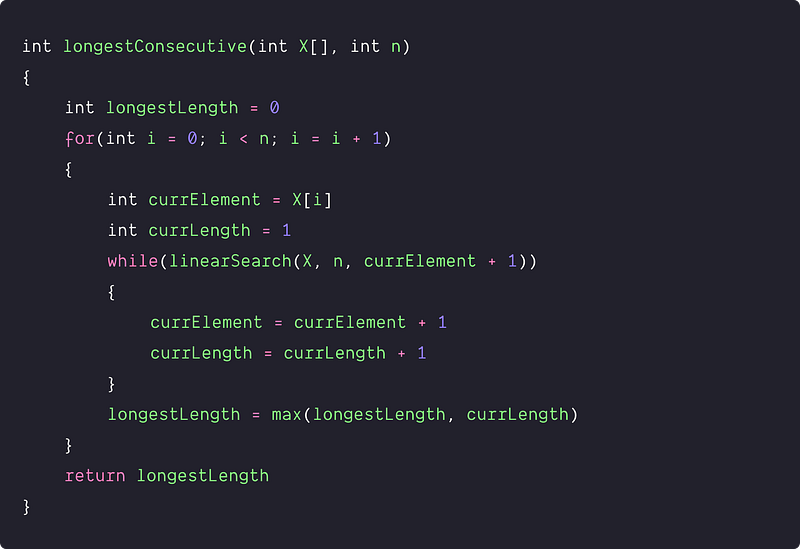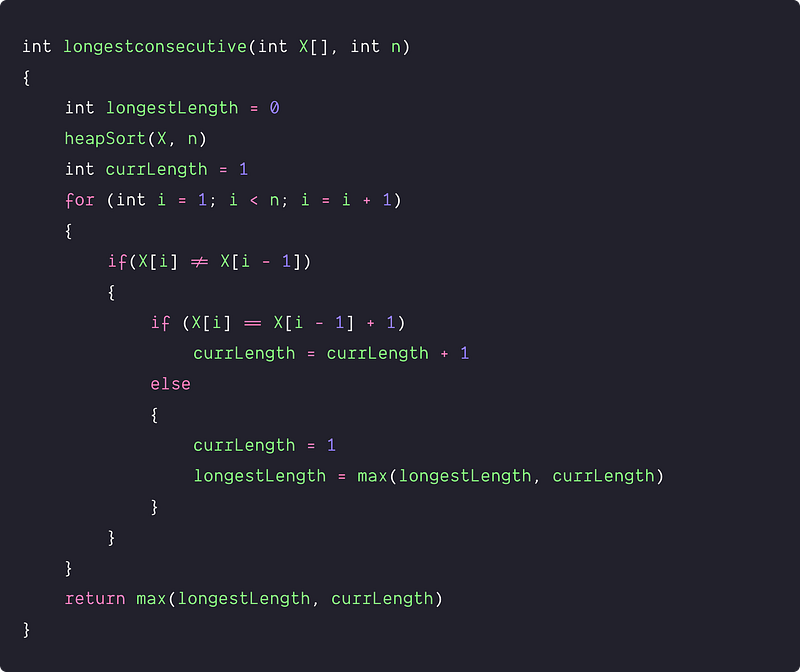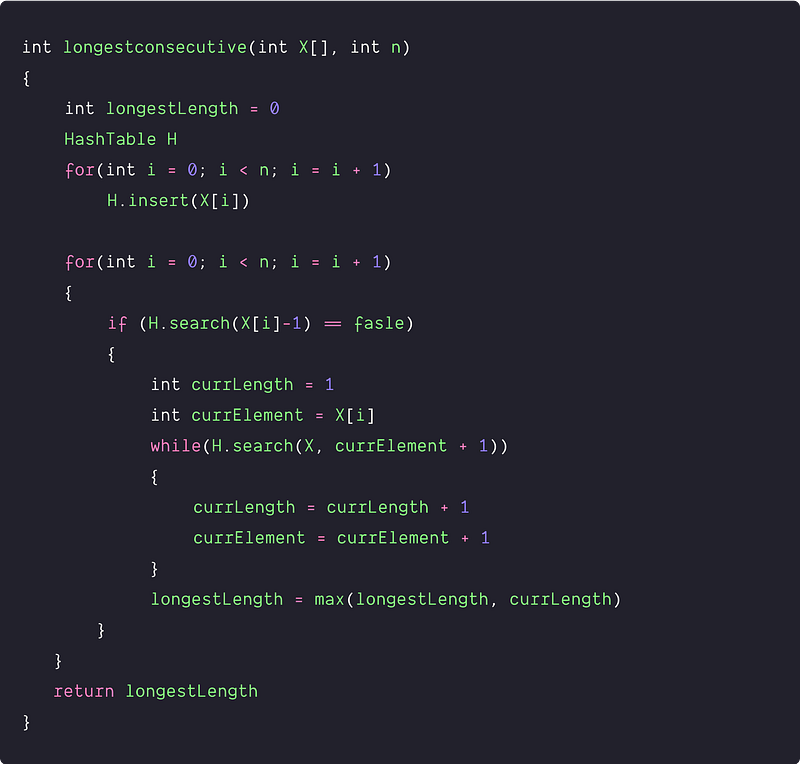# Longest Consecutive Sequence

Key takeaway: One of the best searching problems is learning problem-solving and step-by-step optimization using sorting and hash table.

### Let’s understand the problem

Given an unsorted array X[] consisting of n integers, write a program to find the length of the longest consecutive sequence of integers in the array. The consecutive numbers can be in any order.

Example 1

Input: X[] = [40, 4, 50, 1, 2, 13, 9, 3, 29], Output: 4

Explanation: The longest consecutive sequence is 1,2,3 and 4.

Example 2

Input: X[] = [0, -3, 5, -1, -2, 1], Output: 5

Explanation: The longest consecutive sequence is -2,-1,0,1 and 2.

### Discussed solution approaches

• A brute force approach  using nested loops
• Using sorting and single loop
• An efficient approach using a hash table

### A brute force approach  using nested loops

Solution Idea

The longest consecutive sequence must be starting from some element in the array. So the basic idea would be:

• Pick each element in the input array.
• Perform the linear search to count the length of the longest consecutive elements starting from that element.
• Keep track of the longest length of consecutive elements in the array.

The algorithm is a brute force because it explores every possibility. The critical question is: how do we implement this? Let's think!

Solution Steps

1. We initialize a variable longestLength to track the length of the longest consecutive sequence. longestLength = 0.
2. We run a loop from i = 0 to n-1 to pick each element in the input array. Inside the loop, we initialize two variables: currLength to store the length of the longest consecutive sequence starting from any element X[i] and currElement to track the next consecutive element of the sequence. currElement = X[i], currLength = 1

• Now we search the next element in the sequence (currElement + 1) using the linear search. If the next element is present in the array, we increment the value of currLength by one and move to the next possible consecutive element by incrementing currElement by 1. This process continues in an inner loop until we find an element missing in the consecutive sequence. In this situation, the linear search returns false, and we stop the inner loop.
``````while(linearSearch(X, n, currElement + 1))
{
currElement = currElement + 1
currLength = currLength + 1
}
``````
• By end of the inner loop, if(currLength > longestLength), we update the longestLength with currLength. In other words, the length of consecutive sequence starting from element X[i] is larger than the length of the longest consecutive sequence calculated till that point (elements from X to X[i-1]).
``````if(longestLength < currLength)
longestLength = currLength
``````
3. Now we move to the next iteration of the outer loop to do a similar process and calculate the longest consecutive sequence starting from the element X[i+1].
4. By end of the outer loop, we return the value stored in the variable longestLength.

Solution PseudocodeTime and space complexity analysis

The time complexity of the inner while loop depends on the length of the longest consecutive streak for each element(which is O(n) in the worst case) and the time complexity of the linear search. The time complexity of the inner while loop in the worst case = O(n) * Time complexity of linear search = O(n)*O(n) = O(n²)

Also, this process is repeated for each element of the array. So the overall time Complexity = n * O(n²) = O(n³). In other words, this is a scenario of three nested loops. (Think!)

Space complexity is O(1), as we are using a constant number of variables to generate the output

### Using sorting and single loop

Solution Idea and Steps

Suppose we sort the input array and iterate over each element. In that case, it will be easy to find sequences of consecutive numbers because consecutive elements will be linearly lined up next to each other.

We can use the two variables currLength and longestLength to track the current length of the consecutive sequence and length of the longest consecutive sequence.

• Suppose we are using some efficient in-place sorting algorithm heap sort.
• After sorting, we scan the array and compare each element X[i] to its previous element X[i-1]. If the current and the previous elements are equal, then we simply move to the next element.
• If they are not equal, then we check whether the current element is the next element in the consecutive sequence (i.e. X[i] = X[i-1] + 1). If it does, we increment currLength by one, move to the next iteration, and pick the next element.
• Otherwise, the sequence is broken. We update the value of the longestLength, reset the value of currLength to 1. Now we move to the next iteration and pick the next element in the sorted array.
• By the end of the loop, it is possible that the last element X[n-1] may be part of the longest sequence. In other words, if (X[n-1] == X[n-2] + 1), then X[n-1] is a part of continuous sequence of X[n-2] and currLength get incremented to 1. After this, it will go to the next iteration, the loop will end due to loop condition, and the value of currLength will not be considered for the calculation of longestLength. To handle this situation, by the end of the loop, we need to return the maximum length of the current sequence and the longest one i.e. return max(currLength, longestLength).

Solution PseudocodeTime and space complexity analysis

Time Complexity = Time complexity of sorting + Linear traversal of the array = O(nlogn) + O(n) = O(nlogn)

Space Complexity: If we use heap sort, O(1), else if we use merge sort, O(n).

### An efficient approach  using a hash table

Solution Idea and Steps

In the previous solution, sorting helped us calculate the longest sequence in O(n), but the sorting algorithm still dominates overall time complexity. The critical question is: how can we optimize the time complexity further? Let’s think.

The solution idea is inspired by the idea of the brute force approach. Instead of using linear search to find the next element in the sequence, can we use a hash table for searching? As we know, the hash table does fast searching in O(1) time complexity on average. Think!

According to the problem, there will be two types of elements in the array :

• Type 1: Elements that are starting elements of the consecutive sequence.
• Type 2: Elements that are the intermediate values of some consecutive sequence.

If we know the starting element of any consecutive sequence (Type 1), we can easily calculate the length of the sequence by searching all the next successive elements. So one solution idea is - we need to identify the elements of type 1, count the sequence of length starting from any such element, and return max among them.

If we observe the sorted array approach, we are doing a similar process. When we encounter a different starting element, we reset the sequence length and update the max sequence length. But how do we implement this idea using a hash table? Let's think!

1. We initialize a hash table of size n and a variable longestLength to store the length of the longest consecutive sequence.
2. Now we insert all the elements of the array in the hash table.
3. Now scan each element X[i] using loop and perform the following operations:

• search (X[i] - 1) into the hash table.
• If X[i] - 1 exists in the hash table, it is not the first element of its corresponding sequence. So, we can ignore this and move to the next element.
• If X[i] - 1 does not exist in the hash table, then X[i] is the first element of its corresponding sequence, and we use the similar process used in the brute approach. The only difference here is - we use a hash table instead of a linear search to find the consecutive occurrences.
• Now we calculate the longest consecutive sequence starting from X[i] and store it in the variable currLength. We also update the length of the longest consecutive sequence if currLength > longestLength.
4. By the end of the loop, the value of the longest consecutive sequence gets stored in the variable longestLength. We return this value.

Solution PseudocodeTime and space complexity analysis

At first sight, the time complexity appears to be quadratic due to the two nested loops. But it requires a closer look because while loop is running only when any element X[i] marks the beginning of a sequence. A better idea would be to calculate the count of the critical operations inside the loop to analyze such a situation.

• Searching in the hash table is the critical loop operation.
• In the worst case, each element is searched at most two times: first in the if condition and second in the while loop condition.
• There are n elements in the array and the time complexity of each searching operation is O(1).

Overall time complexity = Time complexity of inserting n elements into hash table + time complexity of searching n elements twice = n*O(1) + 2*n*O(1)= O(n)

Space Complexity = O(n), for the Hash Table

### Critical ideas to think!

• Do the above algorithms work if repeated numbers are in the array?
• Can we think of solving this problem using dynamic programming?
• Can we solve this problem using some other data structures like a heap, BST, etc.? What is the worst-case time complexity if we use BST in place of Hash Table?
• What is the best and worst-case input for all the above approaches?

### Comparison of time and space complexities

• Using nested loops: Time = O(n^3), Space = O(1)
• Using sorting and single loop: Time = O(nlogn), Space = O(1)
• Using a hash table: Time = O(n), Space = O(n)

### Suggested problems to practice

• Find the longest increasing consecutive subsequence
• Sort the numbers in an array based on their frequency
• Given an array of integers, and a number K, print all pairs in the array whose sum is equal to K.
• Find the largest increasing sequence of consecutive positive integers.

Enjoy learning, Enjoy Algorithms!

Get well-designed application and interview centirc content on ds-algorithms, machine learning, system design and oops. Content will be delivered weekly.